All You Need to Know About

# Text Formulas in Excel

Transforming strings in Excel is made fast and easy thanks to the Excel Text Functions. Learn about the different ways you can apply the Text Functions in Excel!

Here are the top things on what you can do with the Text Formulas in Excel:

## How to Remove Leading and Trailing Spaces in Excel

Leading spaces (at the start of the text) and Trailing spaces (at the end of the text) usually get in the way when we want to perform operations in Excel.

Whether it has leading or trailing spaces, we already have a couple ways how to clean them in Excel.

For example using the TRIM formula: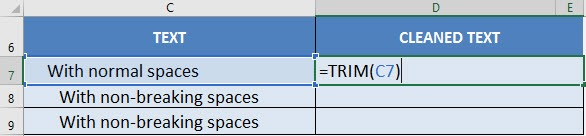The spaces get removed with no issues: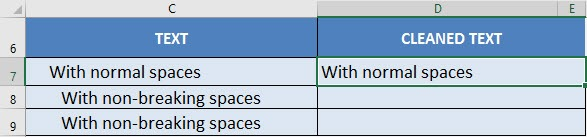However if we have non-breaking spaces, the TRIM formula will not remove these spaces: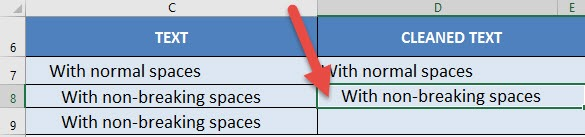The leading space is still there!

What are non-breaking spaces you ask?

Sometimes data downloaded as text uses non-breaking spaces for formatting purposes i.e. it prevents an automatic line break in between these spaces.

This is represented by CHAR(160):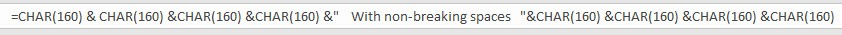This looks like a pain! How do we clean these? I explain how you can do this below:.

STEP 1: We will use the SUBSTITUTE Formula to remove the non-breaking spaces depicted by CHAR(160)

The goal is to replace the non-breaking spaces with normal spaces.

## =SUBSTITUTE(C9, CHAR(160), ” “)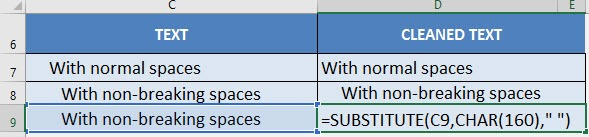STEP 2: Now that we do not have the non-breaking spaces anymore, let us use the TRIM Formula!

## =TRIM(SUBSTITUTE(C9, CHAR(160), ” “))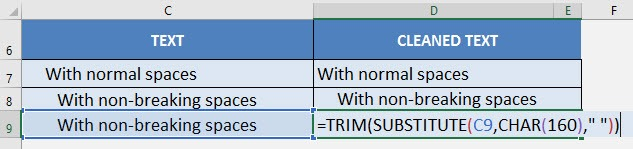Now all of your leading and trailing spaces are cleaned up!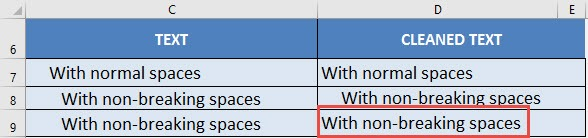## BETWEEN Formula in Excel

There is no explicit Between formula in Excel, however we can come up with creative ways to create this functionality. Our goal is to evaluate if a given value is between a range, for example, is 6 between 1 and 10?

We have three possible scenarios: numbers, dates, and text.

I explain how you can do this in the Between Formula Example below:

STEP 1: For numbers, we have a creative use of the Median formula: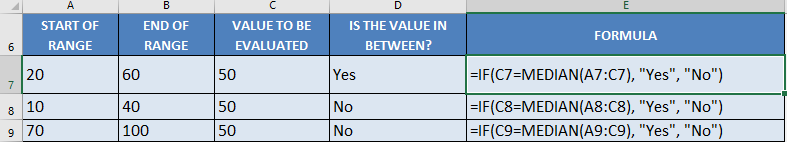## =IF(C7=MEDIAN(A7:C7), “Yes”, “No”)

In our first example above, the range is 20-60, upon checking the value 50, it is in between this range.

The median formula will return the value in the middle of these 3 values when arranged in increasing order: 20, 50, 60. The median value is 50. Since it matches the value we are evaluating, then the answer we get is a Yes, this value (50) is in between the range.

STEP 2: For dates, we have the same application of the median formula. Because Excel treats dates as numbers too:

## =IF(C10=MEDIAN(A10:C10), “Yes”, “No”)

In our first example above, the range is May 1 – July 1, upon checking the date June 1, it is in between this range.

The median formula will return the value in the middle of these 3 dates when arranged in increasing order: May 1, June 1, July 1. The median value is June 1. Since it matches the value we are evaluating, then the answer we get is a Yes, this value (June 1) is in between the range.

STEP 3: For text, we are checking if the value is alphabetically in the middle. We will be using the and formula: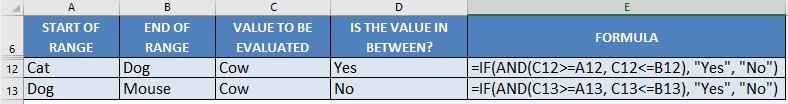## =IF(AND(C12>=A12, C12<=B12, "Yes", "No")

Interestingly enough, you can compare texts using the >= and <= operators.  Excel is able to compare them which goes alphabetically first or last.

In our first example above, the range is Cat – Dog, upon checking the text Cow, it is in between this range. As when arranged alphabetically, it would be: Cat, Cow, Dog.

The And formula checks if Cow >= Cat, and Cow <= Dog. You will see that both of these are true, as Cow is alphabetically later than Cat, while Cow is alphabetically ahead of Dog. Which is why we get a Yes result.

## Concatenate With A Line Break

What does it do?

Joins two or more text strings into one string. The item can be a text value, number, or cell reference.

Formula breakdown:

=CONCATENATE(text1, [text2], [text3], …)

What it means:

=CONCATENATE(the first text, the second text, and so on…)

Excel’s CONCATENATE functions joins two or more text strings into one string.  The item can be a text value, number, or cell reference.

If you add a double quotation with a space in between ” ” then this will add a space between the texts selected on either side.

You can also add a line break in between each text string.  This is done by entering the CHAR(10) function in between each text string/argument.  You will then need to select WRAP TEXT in order to see each text on a separate line.

See how easy this is to implement this by using employee data on the example below.

STEP 1: We need to enter the CONCATENATE function in a blank cell:

## =CONCATENATE(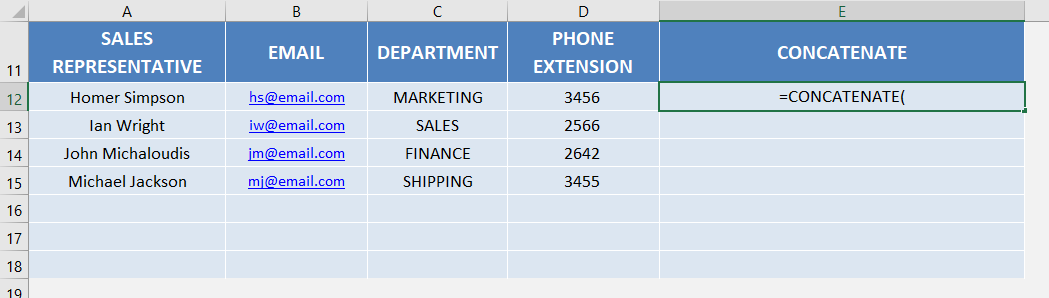STEP 2: The CONCATENATE arguments:

## text1, [text2], [text3], …

Which text do you want to join together?

Let us select all the columns:

## =CONCATENATE(A12, B12, C12, D12)Now let’s add the function CHAR(10) to add a line break between each text

## =CONCATENATE(A12, CHAR(10), B12, CHAR(10), C12, CHAR(10), D12)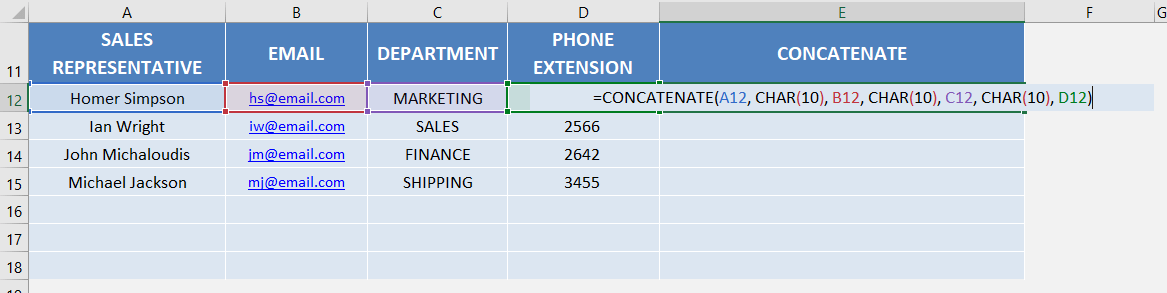Apply the same formula to the rest of the cells by dragging the lower right corner downwards.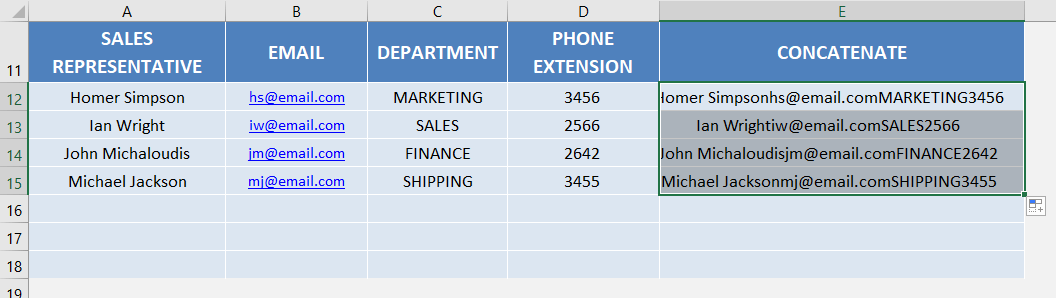STEP 3: Go to Home > Alignment > Wrap Text to show the text in multiple lines and you now have all of results!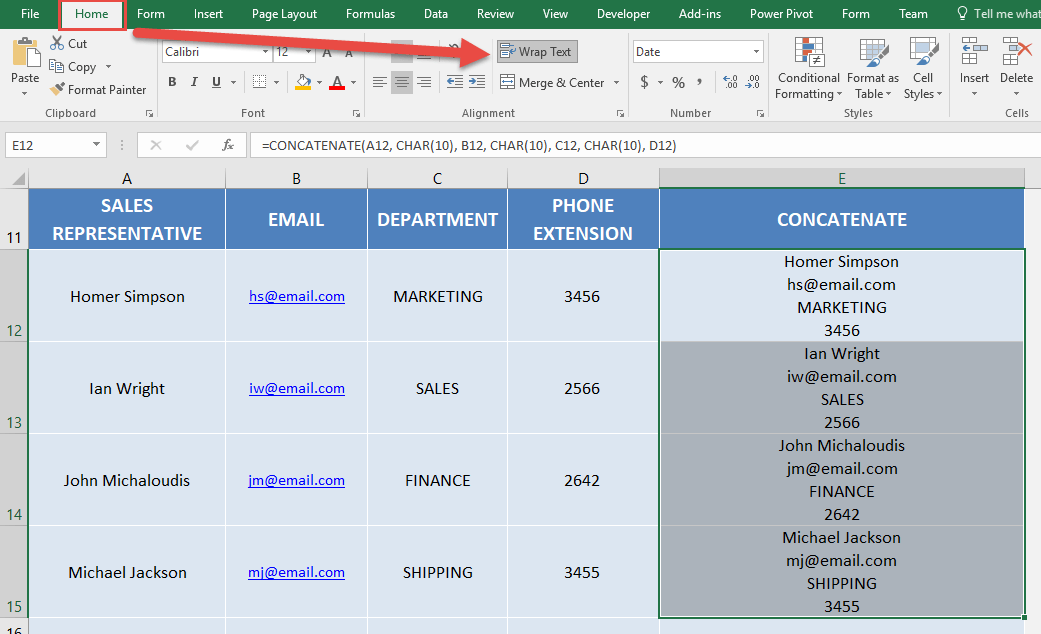Latest Tutorials

July 6, 2021

March 11, 2021

March 3, 2021

January 20, 2021

August 13, 2020

July 11, 2020